# Cayley Graphs

On this page I will discuss some ideas which, while not be immediately useful, are I hope interesting, and may lead to further insight into the theory behind permutation puzzles. My thanks go to Bram Cohen who inspired me to look into this.

## Graphs

A Graph is a set of points (called nodes or vertices), any two of which may or may not be connected by a line. The connecting lines are called the edges of the graph. A graph can be drawn in many ways, as the only thing that is important is which nodes are connected to which. It does not matter how the nodes are arranged on the paper, and while the edges are usually drawn as straight lines, but this is not a necessity. The edges may cross, as long as it is clear that this crossing point is not another node. Below I show some different ways to draw the same graph.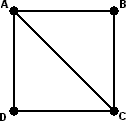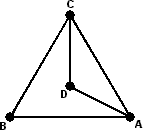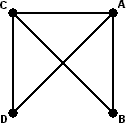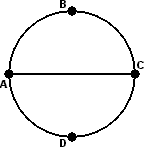## Graphs of puzzles

One example of the use of a graph to solve a puzzle can be seen on the Instant Insanity page. There the puzzle was repackaged in graph form, and from the graph it was much easier to find the solution. Most puzzles that involve matching up patterns can be represented by a graph, but Instant Insanity is one of the few cases where this makes the puzzle significantly simpler. Often it is easier to just try out all the possibilities than it is to build the graph.

Any permutation puzzle can also be represented by a graph. Each node represents one of the possible positions of the puzzle, and two nodes are connected if their positions differ by a single move. Some puzzles such as Atomic Chaos have moves which cannot be undone directly. We could still construct a graph, but its edges must now have arrows on them to show that the move can only be done in one direction. A graph where edges have a direction is called a directed graph.

If we have the whole graph of a puzzle, it becomes obvious what you have to do to solve it. Find the node in the graph that represents the current puzzle position, and the node representing the solved position. Then all you have to do is find some path along the edges of the graph from the current node to the solved node. As you travel along the path of your solution, the puzzle follows suit - each edge corresponds to a move which brings the puzzle to a position represented by the next node on the path. Edge by edge and node by node you get closer to the solved node, just as the puzzle move by move and position by position get closer to being solved.

## Examples

Of course, most permutation puzzles have rather a lot of positions, so the corresponding graph tends to be a bit unwieldy to say the least. Lets therefore construct some extremely simple puzzles and find their graphs to illustrate the ideas so far.

1. Lets consider the squared slice group of the cube. This is what you get if you start with the solved cube, and from there only allow the moves R2L2, F2B2 and U2D2. If you map out the graph of this puzzle, you will soon find that it is in the shape of a cube: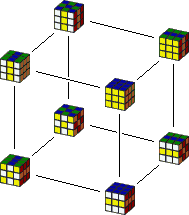2. Consider the puzzle with a track in the shape of a two triangles with a shared edge. The puzzle has four pieces, one at every corner. A move of this puzzle is sliding around the three pieces of one triangle, and so cyclically permuting them. In other words, if the four possible locations of the four pieces are numbered from 1 to 4, the two moves are (123) and (134). The graph looks as follows: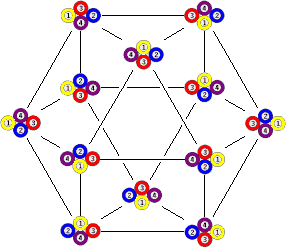3. Now consider the same puzzle as above, but suppose that the track of the left triangle is narrower so that one piece on the right triangle (number 2) will never be able to move around the left triangle (i.e. can't go to position 4). Suppose also that only clockwise cycles are allowed.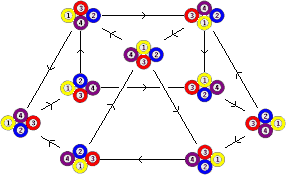This last graph differs from the previous one in two ways. Some nodes (and their edges) are missing, since some positions are no longer possible. Also, all edges now have arrows because the restriction to clockwise moves only makes it impossible to undo a move directly.

## Cayley Graphs

The last example illustrates an important point. In the first two graphs, every node has the same number of edges. This is because every move is possible at every position, and so the number of edges at each node is the same as the number of moves that are possible on the puzzle. In the third example some moves were occasionally blocked. Here the puzzle positions do not form a group (see the simple maths page), and this makes the graph more irregular. If a puzzle has one-way moves, i.e. moves cannot be undone, then the graph will generally also have irregularities.

If moves are never blocked, can always be undone, and all the pieces of the puzzle are different, then the puzzle positions form a group generated by the puzzle's moves. The graph associated with the puzzle is then very regular, and is called the Cayley graph of the group.

It is clear that there is more regularity in a Cayley graph than merely the fact that each node has the same number of edges. The reason for this is simple. Suppose you mix up the puzzle, but then relabel all the pieces so that it is now in the solved position. The puzzle is still the same, so has the same graph as before. Nevertheless, the new solved position actually corresponds to some mixed position originally. You can in fact redraw the graph so that all the edges look the same, except that the node representing the chosen mixed position now in the place where the solved node was. This means that every node in the graph in a sense looks the same, plays the same role in the big picture.

## Uniform Polyhedra

Look again at the first two examples of Cayley graphs. The first one looks like a cube, a Platonic solid, and the second has the shape of a cuboctahedron, an Archimedean solid. The Platonic and Archimedean solids are uniform, that is to say, they have so much rotational symmetry that one vertex can be mapped onto each of the others. As we have just seen, that is exactly the property that a Cayley graph must have.

The (convex) uniform polyhedra consist of the Platonic and Archimedean solids, the prisms, and the anti-prisms. Many of these shapes do actually occur as Cayley graphs, but not all. Here is a short list of uniform shapes, most with a set of group generators that give a Cayley graph of that shape.

 Name Nodes Generators Group Tetrahedron 4 (12)(34), (13)(24), (14)(23) Z2×Z2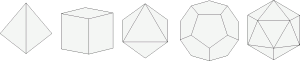Cube 8 (1234), (13) D4 Octahedron 6 (123), (12), (23) D3=S3 Dodecahedron 20 Impossible Icosahedron 12 (123), (234), (13)(24) A4 Truncated Tetrahedron 12 (123), (12)(34) A4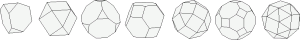Cuboctahedron 12 (123), (234) A4 Truncated Cube 24 (123), (34) S4 Truncated Octahedron 24 (1234), (12) S4 Rhombicuboctahedron 24 (1234), (123) S4 Rhombitruncated Cuboctahedron * 48 (12)(45), (13)(46), (25) Z2×S4 Snub Cuboctahedron 24 (1234), (123), (34) S4 Icosidodecahedron 30 Impossible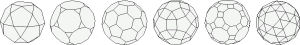Truncated Dodecahedron 60 (124), (23)(45) A5 Truncated Icosahedron 60 (12345), (23)(45) A5 Rhombicosidodecahedron 60 (12345), (124) A5 Rhombitruncated Icosidodecahedron * 120 (1 2)(4 7)(5 11)(9 12), (3 6)(4 5)(7 11)(8 10), (2 3)(4 6)(8 11)(9 10) Z2×A5 Snub Icosidodecahedron 60 (12345), (124), (23)(45) A5 Regular n-gon n (123...n) Zn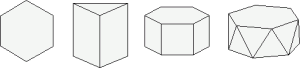Triangular Prism 6 (123), (13) D3=S3 Prism 2n (123..n), (1 n)(2 n-1)(3 n-2)... Dn Anti-prism 2n (12), (345...n+2), (12)(345...n+2) Z2×Zn Pictures made using Poly.

* These two cases were erroneously marked as impossible, but they can be realised using reflections of a cube/octahedron or icosahedron/dodecahedron respectively.

Of course these are all fairly unusual examples of Cayley graphs, because of the mere fact that they correspond to uniform solids. All the graphs in these examples are planar, i.e. can be drawn without having any edges crossing each other. This is because you can enlarge any one face of the solid until it encircles all the rest, and the surface of the solid then becomes (projected on) the flat plane.

Some of these uniform solids can be realised as Cayley graphs from different groups. For example, the prism can be the Cayley graph of Z2×Zn by using generators (12) and (345...n+2). The antiprism can also be the Cayley graph of Z2n, using (123...2n) and (123...2n)2. It turns out that the only groups that can give planar Cayley graphs are exactly those listed above, namely Zn, Z2×Zn, Dn, S4, Z2×S4, A4, A5, and Z2×A5, which are exactly the symmetry groups of the uniform polyhedra. This was proved by Heinrich Maschke in 1896.
(The representation of finite groups. Amer. J. Math. 16 (1896) 156-194.)

Cayley graphs are generally not planar, and most cannot be realised in two or three dimensions with all its edges the same length. Sometimes they are simply one of the above graphs with a full set of diagonals added as edges. For example, the graph generated by (12), (23), and (13) looks like a hexagon with its three long diagonals. It could also be in the shape of some non-convex uniform polyhedron. Despite not being planar graphs most of the symmetry is still visible in these cases. In general the graphs are too intricate for their full symmetry to be captured in two or three dimensional figures. Using more than three dimensions may be possible of course but not as easy to visualise.

## The impossible Dodecahedron

In the list above it states that the dodecahedron does not occur as a Cayley graph. I will give an elementary proof here. It is not necessary to know this proof to understand the rest of this page, but it is included here for completeness.
Each vertex of the dodecahedron has three edges, so if we think of it as a Cayley graph of the group of some puzzle, then there must be three moves in this puzzle. There are two cases to consider:

1. There are two generators, one is its own inverse, and one is not.
2. There are three generators, all are their own inverse.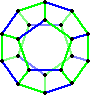Suppose there are two generators, A and B, and B is its own inverse. The moves corresponding to the three edges leaving a node are then A, A', and B. Lets colour all the edges of the dodecahedron corresponding to B blue, and the rest green. Every vertex has exactly one blue edge leading from it, and two green edges. Whatever colouring you choose, the 20 green edges will form loops. These loops represent what happens if you repeatedly apply A (or A'), so they must all be of the same length. It is fairly easy to see that it is not possible to have 4 loops of length 5, or 2 loops of length 10, so the only possible colouring remaining is the one where the green edges form one long loop of 20 edges. There will be some face of the dodecahedron that has only one blue and four green edges, or rather one B and four As. This means that AAAA or A4 has the same effect as B. Then A8 has the same effect as B2, i.e. it has no effect. The green edges don't come in loops of size 8 however, so it is impossible to find an edge colouring that satisfies all the necessary conditions, and there are no such generators A and B.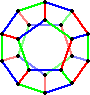Now let's do the other case. Suppose there are three generators, A, B, and C, all their own inverse. The moves corresponding to the three edges leaving any node are then A, B, and C. We could then give the graph a 3-colouring by colouring all A edges red, say, all B edges blue, and all C edges green. Every vertex has all three colours, and there must be 10 edges of each colour. Furthermore, each face must contain all three colours, each colour used exactly once or twice. Focus on the B (blue) edges again. There are too few to occur twice on every face, so there is at least one face with only one blue edge. The edges around this face must correspond to BACAC, in some direction. Therefore B has the same effect as ACAC. For the same reason there must be a face with only one A (red) edge, and this will correspond to BACBC, so B must also have the same effect as ACBC. Combining these we get ACAC=ACBC, so A=B. This is not possible, so the case of three generators fails too.

## Distances

The distance between two puzzle positions is the least number of moves you need to get from one to the other. Similarly, the distance between two nodes in a (Cayley) graph is the least number of edges you have to traverse to get from one node to the other. In general this is a rather hard problem because there are so many paths/move-sequences that are possible, and not only must they connect the two nodes/positions, they must be the shortest possible. In the simple cases of the uniform polyhedra it is easy to find distances just by inspecting the shape. You can just follow the edges that go to the end node in as straight a line as possible, and it will be a shortest path. Without such visual cues, this is hard.

Suppose you have a (Cayley) graph made out of string - each node is a knot, and the edges are strings of all the same length. This would look a bit like one of those net bags that oranges are sold in. If you hold it at one node and let it dangle, then all the adjacent nodes will hang at the same height just below the top node. All nodes at distance two will hang at a level just below that, nodes at distance three below that, and so on. The node(s) furthest away will hang furthest down.

In general graphs the diameter is defined as the distance between two nodes that are furthest apart. Cayley graphs are uniform, so this dangling string net will look exactly the same regardless of which node you picked it up at. In particular, the diameter is the distance between the top and bottom of this dangling net.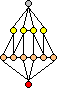Let's push this dangling net bag visualisation a bit further. When you pick it up, most of the material hangs as a bunch near the bottom. It is like a teardrop shape. This observation matches what is shown by calculations of permutation puzzles. The number of puzzle positions increases by a fairly steady factor every step further away from the start position, until most puzzle positions have been reached. After this the number of positions further out decreases drastically within a few steps. The distribution of positions at each distance is similar to the thickness of the net bag at each height.
On the right you see an example, which is same graph as the cuboctahedron in example 2 above (except that two horizontal edges have been removed for clarity). In this case the diameter is 3, and there is only one antipode, one position at the furthest distance.

Unlike the previous example, there may be several antipodes. One obvious example we have seen so far is the tetrahedron-shaped graph. Whichever node you pick, the other three are all at distance 1 and are all antipodes. The tetrahedron is unusual in that it has a face opposite each vertex, whereas all other Archimedean solids have faces opposite each other. Prisms with an odd number of sides always have two antipodes, since there is an edge on the opposite side of each vertex.

If a puzzle itself has a certain amount of symmetry, this will show itself in the Cayley graph. The puzzle in example 2 is the same when rotated through 180 degrees. Turns of the left triangle become turns of the right triangle and vice versa, so the set of all allowed moves remains the same. It can also be reflected horizontally or vertically. These symmetries show in the Cayley graph in the fact that the cuboctahedron has rotational and mirror symmetry around an axis through a vertex.
This has implications for a unique antipode. If the starting position is rotated or reflected and then relabeled so that it remains the same, then the antipode must remain the same also if it is rotated/reflected and given the same re-labelling. In the puzzle of example 2 there is only one reachable position that has those symmetries, so we can deduce the antipode without even working out the whole graph. This presupposes however that there is only a single antipode.

Assume for a moment that the Rubik's cube has only one antipode. The cube has lots of symmetry, and there are very few patterns on the cube that exhibit the same amount of symmetry. Other than the solved position, there are only three:

1. The Pons Asinorum (i.e. the 6X pattern or order 2)
R2L2 F2B2 U2D2 (6f*, 12q*)
2. The Superflip
RL D2 B' L2 F2 R2 U'D R' D2 F'B' D' F2 D' R2 U' F2 D' (20f*, 28q)
R' U2 B L' F U' B D F UD' L D2 F' R B' D F' U' B' UD' (22f, 24q*)
3. The above two patterns combined.
B' D' L' F' D' F'B U F'B R2 L UD' F L U R D (19f*, 20q*)

The Superflip is the furthest of these three from start, so if there is a single antipode, that must be it. If half turns are counted as one move, then it is at distance 20, and if only quarter turns are counted as moves, then at distance 24. There is however one position known to be 26 quarter turns from start:

1. The 4-spot combined with superflip
U2 D2 L F2 U' D R2 B U' D' R L F2 R U D' R' L U F' B' (21f, 26q*)
F U2 R L D F2 U R2 D F2 D F' B' U2 L F2 R2 B2 U' D (20f*, 28q)

This proves that in the quarter turn metric, there is no unique antipode because the fully symmetric positions are not antipodes. This last pattern is the furthest known so far, and if it is indeed an antipode then there are at least three, because the pattern can occur in three different orientations.
Similarly, in the half turn metric there are other positions that are 20 moves away from start, showing that there is no unique antipode there either.

## Identities

A walk (or circuit) on a graph is some path of edges that brings you back to the node you started from. If you picture an Archimedean solid, a walk on its edges can be long and meandering, even intersect itself, or it can be very short and just encircle one face. It is obvious that for any two nodes there is a walk that visits them both, because the graph is connected and the walk can simply retrace its steps. A Cayley graph has enough edges however that there will always be a walk visiting those two nodes and which does not visit any node twice. It is much harder to determine whether or not there is a walk that visits every node exactly once, a Hamiltonian circuit.

Two walks that have a node in common can be joined together - starting from the shared node first follow one walk and then follow the other. If the two walks share not just a single node but an edge, then we could remove that edge from both walks when we join them together. For example a walk around one face and a walk around an adjacent face becomes a walk around the outside of both faces joined together, and we don't bother to go up and down the shared edge.
A walk on a Cayley graph of a puzzle corresponds to some sequence of moves that has no effect, i.e. an identity. Just like two walks can be joined, two identities can be joined by applying one after the other. If the last moves of the first identity are immediately cancelled by the second identity, this is the same as removing a shared edge when joining two walks.

Consider any Archimedean solid. It is a uniform shape, so the face arrangement around each vertex is the same. So if we know which faces meet at each vertex, and in which order, then the shape of the whole solid can be deduced. In the same way a whole Cayley graph can be deduced once the arrangement of faces at one node is known. But what exactly is a face in such a graph? Without a surface to guide us (such as the outside of a solid), there is not always a clear choice as to what constitutes a face.
A walk on the edges of an Archimedean solid can go around several faces, and in a similar way any identity of a puzzle can correspond to a walk in its Cayley graph which goes around one or more faces. Clearly if a walk can be built from two or more shorter walks, then it does not go around a single face. If we choose some set of walks such that any others can be built from shorter walks in our set, then we can say that our set of walks define the faces of the Cayley graph. Of course we would like this set to contain as few walks as possible, so it should not contain any walks which themselves can be built from shorter ones in the set.

The similarity between our set of faces on a general Cayley graph and the faces of an Archimedean solid breaks down slightly. In the general Cayley graph we might have two faces that share more than one edge, or an edge that is shared by more than two faces. For one extreme example, consider the normal Rubik's Cube. The following three move sequences all have the same effect:
a. FU'R'FRF'
b. U'FRU'R'U
c. UL'U'LFU'
This gives rise to three identities of 12 quarter turns, ab', bc', and ca'. There aren't any shorter identities on the cube (except for trivial ones), so these are three faces, each sharing half its edges with the other two. Although any one of them is the result of joining the other two, they do not split into shorter identities, so they are all considered faces in the graph. We can say that faces correspond to irreducible identities, i.e. identities that cannot be made by joining smaller ones.

If we know all the faces at a vertex of a uniform solid, then we can map out the whole thing. Similarly if we somehow manage to find all irreducible identities of a puzzle, the whole puzzle is theoretically known. It could therefore be used to work out the diameter of the group, or more generally, the number of positions at each distance from start. In practice this is not simple as the sheer size of the group may make it too hard to work out. If the number of short irreducible identities is relatively small, then this way of looking at the group might lead to a more feasible enumeration method up to some depth than the standard breadth first search. There are generally too many longer irreducible identities for this approach to work all the way to the antipode.

Suppose you were doing a breadth first search of a puzzle. In other words, you construct a list of all positions at distance n+1 by trying out all moves on positions at distance n, and discarding any resulting positions that had already been encountered at distance n or n-1. If you think back to the dangling orange net analogy, you are just building this net node by node, layer by layer from top to bottom. Sometimes you will find that a new position can be reached in several ways, is connected to the previous layer by several edges. This is due to some identity, as the tying together of two edges to the new node makes a 'face' in the graph.

## Example

Let's look for example at the two-generator group <U,R> on the normal Rubik's Cube. There are 6 possibilities for the first move (U,U',U2 and R,R',R2), so there are 6 positions at depth 1. The next move must be the other face, so it has only 3 possibilities, and this holds for all subsequent moves too. You would therefore expect the number of positions at depth n to be 6·3n-1. This is true for a while, but obviously the number of reachable positions cannot increase without bound. The table below shows the whole list:

DistancePositionsTotal
011
167
21825
35479
4162241
5486727
61,4572,184
74,3606,544
813,01619,560
938,48258,042
10113,094171,136
11328,920500,056
12942,3511,442,407
132,616,9734,059,380
146,774,84810,834,228
1515,105,59225,939,820
16    24,231,019    50,170,839
1719,421,27469,592,113
183,843,56873,435,681
1947,46573,483,146
205473,483,200

Up to depth 5, each step has triple the number of new positions. At depth 6 however there is one fewer, namely 1,457 instead of 1,458. This is due to the identity (R2U2)6. The position reached by (R2U2)3 can also be reached by (U2R2)3, so that accounts for the discrepancy. Each further step the number of positions is similarly reduced by other identities. If it were known beforehand that this length 12 identity was the only one up to that length, then the number of positions up to distance 6 can be immediately deduced. After that things get more complicated very quickly, though a computer might be able track of which identities have effect and how much.

The problem with this example is that the identities are mostly rather long. This not only means that there will be very many irreducible ones, it also means that a search for such identities is as difficult if not more so than calculating through the whole group directly.

## Application

Clearly these ideas were not very effective for fully analysing the previous example. We would prefer to have an example where all the irreducible identities are short. This would be the case if moves commute, giving identities of the form ABA'B'. If however all moves commute, then the puzzle is much more easily analysed by linear algebra and combinatorial methods. Examples of that kind are the Lights Out puzzles and Rubik's Clock. The non-commuting moves should also be of low order, to keep the number of identities down. If A and B have orders n and m then there will be at least nm irreducible identities, namely those beginning with AxBy, though some may correspond to the same face of the graph (i.e. the identities are the same up to cyclic rearrangement and/or inversion). Suppose therefore that we have a puzzle
- which has not very many kinds of moves
- of which all moves are of low order
- of which most but not all moves commute
- and which is a bit too large to analyse with the traditional methods.

There are very few puzzles on this site that fit the criteria above. The Gripple or Cmetrick might be a reasonable candidates, provided their symmetries can be used to simplify the problem. I have not yet analysed these puzzles in this way, so I do not know for certain whether the method outlined below is feasible or not.

We would have to find as many irreducible identities as we can. This is best done by an IDA* search, as explained on the Computer Cubing page. Instead of using IDA* to solve a mixed position, we are now using it to 'solve' the starting position. It is not immediately clear when all the irreducible identities have been found so just stop when it seems that there will not be any more.

Once we think all the irreducible identities are known, we have to (get the computer to) construct the graph. Begin with a single node representing the solved position. Do each possible move to make a list of the adjacent nodes at distance 1. For each node you keep track of where it lies with respect to its adjacent faces. Any nodes which are exactly alike in their face arrangements should be collated, i.e. only be stored in memory once with a number indicating how many such nodes there are. From these nodes again all moves are done to create a list of nodes one step further from solved. As all the adjacent faces are known, it is possible to deduce when there multiple ways occur to reach the same node. Again collate any nodes that have identical face arrangements. These steps are repeated until no new nodes can be found.

If all the irreducible identities were found then - assuming there were no mistakes in the rest of the analysis - the total number of nodes will match the number of positions of the puzzle. If the graph grows too large, then some identities must have been overlooked.

The advantage of this method lies in the fact that similar nodes are collated. If there are enough of such nodes, then the memory requirements may be less than in the traditional breadth first search. This may be especially true in cases where the shortest way to solve part of a puzzle will not disturb other parts, and for this reason I suspect that CMetrick is the most logical candidate for this approach. If anyone manages to analyse it in this way, please let me know.